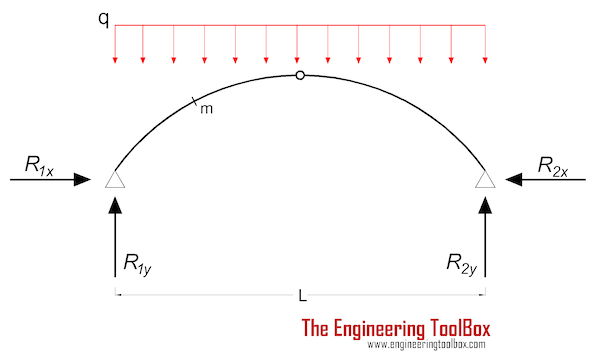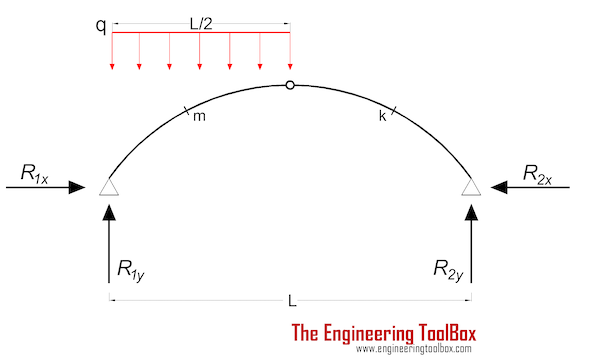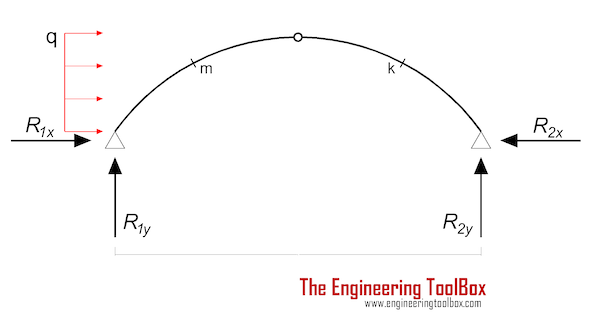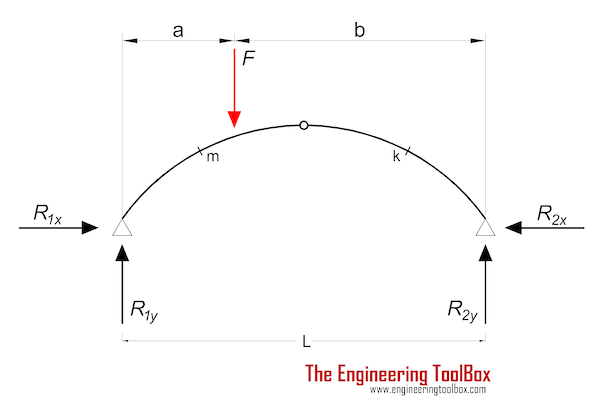Engineering ToolBox - Resources, Tools and Basic Information for Engineering and Design of Technical Applications!

# Three-Hinged Arches - Continuous and Point Loads

## Support reactions and bending moments.

### Three-Hinged Arch - Continuous Load

####Bending Moment

Mm = (q L2 / 8) (4 (xm / L - (xm / L)2) - ym / yc)                               (1a)

where

Mm = moment at m (Nm, lbf ft)

q = continuous load (N/m, lbf/ft)

xm = x-coordinate for m (m, ft)

ym = y-coordinate for m (m, ft)

yc = y-coordinate for center hinge (m, ft)

L = horizontal distance between the supports (m, ft)

Cartesian coordinates related to a center located in the hinge of support no. 1.

#### Support Reactions

R1y = R2y

= q L / 2                                (1b)

where

R = support force (N, lbf)

R1x = R2x

= q L2 / (8 yc)                               (1c)

### Three-Hinged Arch - Half Continuous Load

####Bending Moment

Mm = (q L2 / 16) (8 (xm / L - (xm / L)2) - 2 xm / L  - ym / yc)                               (2a)

#### Support Reactions

R1y = 3 q L / 8                                (2b)

R2y = q L / 8                                (2c)

R1x R2x

= q L2 / (16 yc)                               (2d)

### Three-Hinged Arch - Horizontal Continuous Load

####Bending Moment

Mm = (q L2 / 2) (xm / L - 3 xm / L + (xm / L)2)                                (3a)

Mk = (q L2 / 4) (2 (L - xk) / L - yk / yc)                                (3b)

where

Mk = moment at k (Nm, lbf ft)

yk = y-coordinate for k (m, ft)

xk = x-coordinate for k (m, ft)

#### Support Reactions

R1y = - q yc2 / (2 L)                                (3c)

R2y = q yc2 / (2 L)                                (3d)

R1x = - 3 q yc / 4                               (3e)

R2x = q yc / 4                               (3f)

### Three-Hinged Arch - Eccentric Point Load

####Bending Moment

Mm = (F a / 2) (2 (b / a) (xm / L) - ym / yc)                                (4a)

Mk = (F a /2) (2 (L - xk) / L - yk / yc)                                (4b)

#### Support Reactions

R1y = F b / L                                (4c)

R2y = F a / L                                 (4d)

R1x = R2x

= F a / (2 yc)                               (4f)

## Related Topics

• ### Beams and Columns

Deflection and stress, moment of inertia, section modulus and technical information of beams and columns.
• ### Mechanics

Forces, acceleration, displacement, vectors, motion, momentum, energy of objects and more.
• ### Statics

Loads - forces and torque, beams and columns.

## Related Documents

• ### Area Moment of Inertia - Typical Cross Sections I

Typical cross sections and their Area Moment of Inertia.

• ### Cantilever Beams - Moments and Deflections

Maximum reaction forces, deflections and moments - single and uniform loads.
• ### Continuous Beams - Moment and Reaction Support Forces

Moments and reaction support forces with distributed or point loads.
• ### Euler's Column Formula

Calculate buckling of columns.
• ### Mass Moment of Inertia

The Mass Moment of Inertia vs. mass of object, it's shape and relative point of rotation - the Radius of Gyration.
• ### Stiffness

Stiffness is resistance to deflection.
• ### Structural Lumber - Properties

Properties of structural lumber.
• ### Young's Modulus, Tensile Strength and Yield Strength Values for some Materials

Young's Modulus (or Tensile Modulus alt. Modulus of Elasticity) and Ultimate Tensile Strength and Yield Strength for materials like steel, glass, wood and many more.

## Engineering ToolBox - SketchUp Extension - Online 3D modeling!

Add standard and customized parametric components - like flange beams, lumbers, piping, stairs and more - to your Sketchup model with the Engineering ToolBox - SketchUp Extension - enabled for use with older versions of the amazing SketchUp Make and the newer "up to date" SketchUp Pro . Add the Engineering ToolBox extension to your SketchUp Make/Pro from the Extension Warehouse !

We don't collect information from our users. More about

## Citation

• The Engineering ToolBox (2006). Three-Hinged Arches - Continuous and Point Loads . [online] Available at: https://www.engineeringtoolbox.com/three-hinged-arches-d_512.html [Accessed Day Month Year].

Modify the access date according your visit.

9.19.12